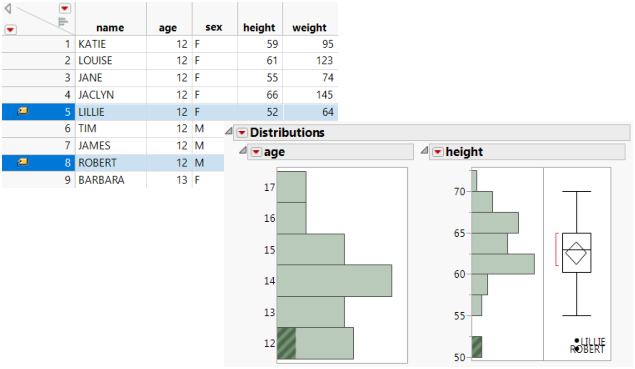Basic Analysis > Distributions
Publication date: 11/10/2021

# Distributions

##### Using the Distribution Platform

Use the Distribution platform to explore the distribution of a single variable using histograms, box plots, and summary statistics. You can perform several types of hypothesis tests, including t tests, z tests, Chi-squared tests, and equivalence tests. You can also construct confidence, tolerance, and prediction intervals, and estimate process capability. The word univariate simply means involving one variable instead of two (bivariate) or many (multivariate). However, you can examine the distribution of several individual variables within a report. The report content for each variable depends on whether the variable is categorical (nominal or ordinal) or continuous.

The Distribution report window is interactive. Clicking on a histogram bar highlights the corresponding data in any other histograms and in the data table.

Figure 3.1 Example of the Distribution Platform